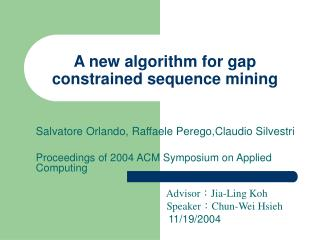DownloadDownload PresentationA new algorithm for gap constrained sequence mining

# A new algorithm for gap constrained sequence mining

Télécharger la présentation## A new algorithm for gap constrained sequence mining

- - - - - - - - - - - - - - - - - - - - - - - - - - - E N D - - - - - - - - - - - - - - - - - - - - - - - - - - -
##### Presentation Transcript

1. A new algorithm for gap constrained sequence mining Salvatore Orlando, Raffaele Perego,Claudio Silvestri Proceedings of 2004 ACM Symposium on Applied Computing Advisor：Jia-Ling Koh Speaker：Chun-Wei Hsieh 11/19/2004

2. Problem • A sequence occurs in under the minimum gap and maximum gap constraints, denoted as , if there exists integers such that , and

3. Min_gap constraint: • Let be an input database sequence. • If , then all its subsequences , , satisfy • . • Min_gap constraint is an anti-monotone constraint

4. Max_gap constraint: • Let be an input database sequence. • If , • then all its subsequences , , satisfy • . ? • Max_gap constraint is not an anti-monotone constraint

5. SPADE • A candidate k-sequence is made by a pair of frequent (k-1) –subsequences that share a common (k-2)-preffix. • . SPADE might loose candidates

6. Contiguous sequences 1. is obtained from by dropping an item from either or ; 2. is obtained from by dropping an item from , where ; 3. is a contiguous subsequence of , and is a contiguous subsequence of .

7. Prefix and Suffix Subsequence • Max_gap constraint becomes anti-monotone, when using contiguous subsequence. • A prefix or suffix of a sequence is a particular contiguous subsequence of

8. cSPADE • cSPADE solves the problem by using the contiguous subsequence concept. • It combines the (k-1)-prex and 2-sux of are contiguous subsequences of • . cSPADE destroys the prex-class

9. CCSM 1) Count-based phase: scanning the database and mining the and 2)The horizontal database is transformed into a vertical one. 3) Intersection-based phase: generating the candidate k-sequence by merging with such that

10. Candidate generation Figure 1: CCSM candidate generation.

11. Idlist intersection • To determine the support of a candidate k-sequence p, we have first to produce the associated idlist L(p). • , , and can be joined to produce • .

12. Idlist intersection Order • . • Left to right : store the eid of the last item/event • Right to left : store the eid of the first item/event • (sid,eid) (sid, first_eid,last_eid)

13. Idlist caching Figure 2: Example of cache usage. Figure 3: CCSM idlist reuse.

14. Experiment 1 Figure 4: Number of intersection operations actually performed using 2-ways, pure k-ways and cached k-waysintersection methods while mining two synthetic datasets.

15. Experiment 2 Figure 5: Number of frequent sequences in datasets CS11 (minsup=0:30) and CS21(minsup=0:40) as afunction of the pattern length for dierent values of the max gapconstraint.

16. Experiment 3 Figure 6: Execution times of CCSM and cSPADE on datasets CS11 (minsup=0:30) and CS21 (minsup=0:40)as a function of the max gap value.

17. Experiment 4 Figure 7: Execution times of CCSM and cSPADE on datasets CS11 and CS21 with a xed max gap constraint(max gap=8) as a function of the minimum support threshold.